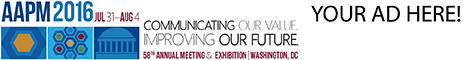# Program Information

## T-Test Based Prior Error Estimate and Stopping Criterion for Monte Carlo Dose Calculation in Proton TherapyX Hong

## Presentations

#### SU-E-T-769 (Sunday, July 12, 2015) 3:00 PM - 6:00 PM Room: Exhibit Hall

Purpose:The Monte Carlo (MC) method is a gold standard for dose calculation in radiotherapy. However, it is not a priori clear how many particles need to be simulated to achieve a given dose accuracy. Prior error estimate and stopping criterion are not well established for MC. This work aims to fill this gap.

Methods:Due to the statistical nature of MC, our approach is based on one-sample t-test. We design the prior error estimate method based on the t-test, and then use this t-test based error estimate for developing a simulation stopping criterion. The three major components are as follows.
First, the source particles are randomized in energy, space and angle, so that the dose deposition from a particle to the voxel is independent and identically distributed (i.i.d.).
Second, a sample under consideration in the t-test is the mean value of dose deposition to the voxel by sufficiently large number of source particles. Then according to central limit theorem, the sample as the mean value of i.i.d. variables is normally distributed with the expectation equal to the true deposited dose..
Third, the t-test is performed with the null hypothesis that the difference between sample expectation (the same as true deposited dose) and on-the-fly calculated mean sample dose from MC is larger than a given error threshold, in addition to which users have the freedom to specify confidence probability and region of interest in the t-test based stopping criterion.

Results: The method is validated for proton dose calculation. The difference between the MC result based on the t-test prior error estimate and the statistical result by repeating numerous MC simulations is within 1%.

Conclusion:The t-test based prior error estimate and stopping criterion are developed for MC and validated for proton dose calculation.

Funding Support, Disclosures, and Conflict of Interest: Xiang Hong and Hao Gao were partially supported by the NSFC (#11405105), the 973 Program (#2015CB856000) and the Shanghai Pujiang Talent Program (#14PJ1404500).

Contact Email: